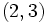# Pronormality is not commutator-closed

This article gives the statement, and possibly proof, of a subgroup property (i.e., pronormal subgroup) not satisfying a subgroup metaproperty (i.e., commutator-closed subgroup property).
View all subgroup metaproperty dissatisfactions | View all subgroup metaproperty satisfactions|Get help on looking up metaproperty (dis)satisfactions for subgroup properties
Get more facts about pronormal subgroup|Get more facts about commutator-closed subgroup property|

## Statement

It is possible to have a group$G$ and pronormal subgroups$H, K$ of$G$ such that the commutator$[H,K]$ is also a pronormal subgroup.

## Proof

### Example of the symmetric group of degree four

Further information: symmetric group:S4

Let$G$ be the symmetric group on the set$\{1,2,3,4 \}$. Let$H = K$ be a$2$-Sylow subgroup of$G$, say:$H = \{ (), (1,2,3,4), (1,3)(2,4), (1,4,3,2), (1,3), (2,4), (1,2)(3,4), (1,4)(2,3) \}$.

Then,$H$ is isomorphic to a dihedral group of order eight.$[H,K] = [H,H] = \{ (), (1,3)(2,4) \}$.

•$H$ is pronormal in$G$: In fact,$H$ is a Sylow subgroup of$G$, and Sylow implies pronormal.
•$[H,H]$ is not pronormal in$G$: This subgroup is conjugate to$\{ (), (1,2)(3,4) \}$ by the permutation$(2,3)$, but these two subgroups are not conjugate in the subgroup they generate.